# Boolean Expression ⁄ Function

Boolean algebra deals with binary variables and logic operation. A Boolean Function is described by an algebraic expression called Boolean expression which consists of binary variables, the constants 0 and 1, and the logic operation symbols. Consider the following example.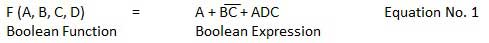Here the left side of the equation represents the output Y. So we can state equation no. 1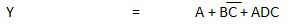## Truth Table Formation

A truth table represents a table having all combinations of inputs and their corresponding result.

It is possible to convert the switching equation into a truth table. For example, consider the following switching equation.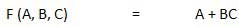The output will be high (1) if A = 1 or BC = 1 or both are 1. The truth table for this equation is shown by Table (a). The number of rows in the truth table is 2n where n is the number of input variables (n=3 for the given equation). Hence there are 23 = 8 possible input combination of inputs.## Methods to simplify the boolean function

The methods used for simplifying the Boolean function are as follows −

• Karnaugh-map or K-map, and
• NAND gate method.

## Karnaugh-map or K-map

The Boolean theorems and the De-Morgan's theorems are useful in manipulating the logic expression. We can realize the logical expression using gates. The number of logic gates required for the realization of a logical expression should be reduced to a minimum possible value by K-map method. This method can be done in two different ways, as discussed below.

### Sum of Products (SOP) Form

It is in the form of sum of three terms AB, AC, BC with each individual term is a product of two variables. Say A.B or A.C etc. Therefore such expressions are known as expression in SOP form. The sum and products in SOP form are not the actual additions or multiplications. In fact they are the OR and AND functions. In SOP form, 0 represents a bar and 1 represents an unbar. SOP form is represented by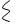.

Given below is an example of SOP.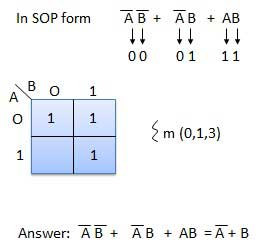### Product of Sums (POS) Form

It is in the form of product of three terms (A+B), (B+C), or (A+C) with each term is in the form of a sum of two variables. Such expressions are said to be in the product of sums (POS) form. In POS form, 0 represents an unbar and 1 represents a bar. POS form is represented by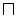.

Given below is an example of POS.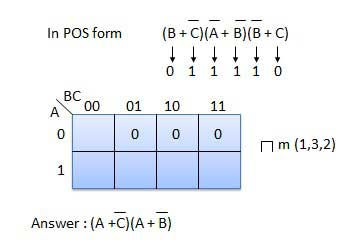## NAND gates Realization

NAND gates can be used to simplify Boolean functions as shown in the example below.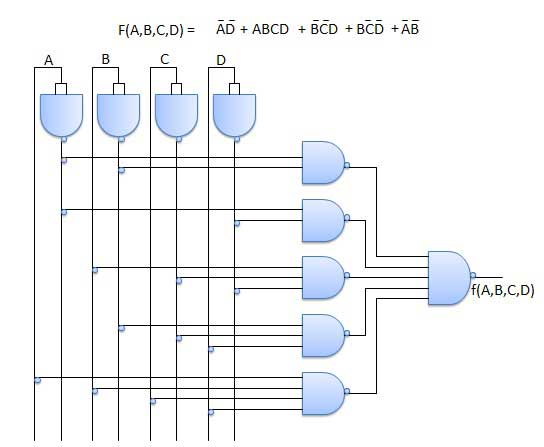boolean_algebra.htm